# Dividing whole numbers by unit fractions

##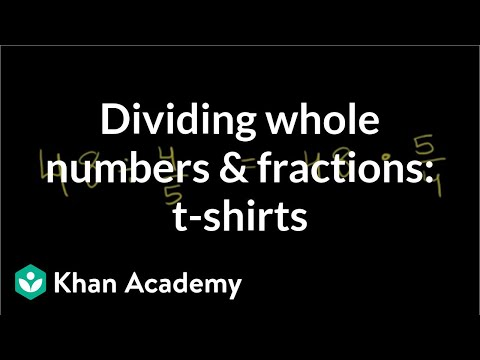By Khan Academy

Dividing a whole number by a fraction? Turn the tables and multiply by the reciprocal of the fraction. Boom!# Dividing whole numbers by unit fractions

##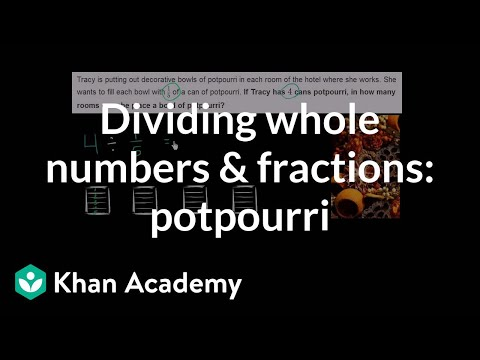By Khan Academy

Solve a word problem by dividing a whole number by a fraction. The example used in this video is 4 ï¿½ï¿½ï¿½ï¿½ï¿½ï¿½ï¿½ï¿½ï¿½ï¿½ï¿½ï¿½ 1/5.# Dividing unit fractions by whole numbers

##By Khan Academy

Solve a word problem by dividing a fraction by a whole number. The example used in this video is 1/5 ï¿½ï¿½ï¿½ï¿½ï¿½ï¿½ï¿½ï¿½ï¿½ï¿½ï¿½ï¿½ 4.# Dividing whole numbers by unit fractions

##By Khan Academy

Dividing a whole number by a fraction? Turn the tables and multiply by the reciprocal of the fraction. Boom!# Dividing whole numbers by unit fractions

##By Khan Academy

Dividing a whole number by a fraction? Turn the tables and multiply by the reciprocal of the fraction. Boom!# Dividing whole numbers by unit fractions

##By Khan Academy

Dividing a whole number by a fraction? Turn the tables and multiply by the reciprocal of the fraction. Boom!# Dividing whole numbers by unit fractions

##By Khan Academy

Solve a word problem by dividing a whole number by a fraction. The example used in this video is 4 ï¿½ï¿½ï¿½ï¿½ï¿½ï¿½ï¿½ï¿½ï¿½ï¿½ï¿½ï¿½ 1/5.# Dividing whole numbers by unit fractions

##By Khan Academy

Solve a word problem by dividing a whole number by a fraction. The example used in this video is 4 ï¿½ï¿½ï¿½ï¿½ï¿½ï¿½ï¿½ï¿½ï¿½ï¿½ï¿½ï¿½ 1/5.# Dividing unit fractions by whole numbers

##By Khan Academy

Solve a word problem by dividing a fraction by a whole number. The example used in this video is 1/5 ï¿½ï¿½ï¿½ï¿½ï¿½ï¿½ï¿½ï¿½ï¿½ï¿½ï¿½ï¿½ 4.# Dividing unit fractions by whole numbers

##By Khan Academy

Solve a word problem by dividing a fraction by a whole number. The example used in this video is 1/5 ï¿½ï¿½ï¿½ï¿½ï¿½ï¿½ï¿½ï¿½ï¿½ï¿½ï¿½ï¿½ 4.# Dividing whole numbers by unit fractions

##By Khan Academy

Solve a word problem by dividing a whole number by a fraction. The example used in this video is 4 ï¿½ï¿½ï¿½ï¿½ï¿½ï¿½ï¿½ï¿½ï¿½ï¿½ï¿½ï¿½ 1/5.# Dividing unit fractions by whole numbers

##By Khan Academy

Solve a word problem by dividing a fraction by a whole number. The example used in this video is 1/5 ï¿½ï¿½ï¿½ï¿½ï¿½ï¿½ï¿½ï¿½ï¿½ï¿½ï¿½ï¿½ 4.# Dividing a whole number by a fraction

##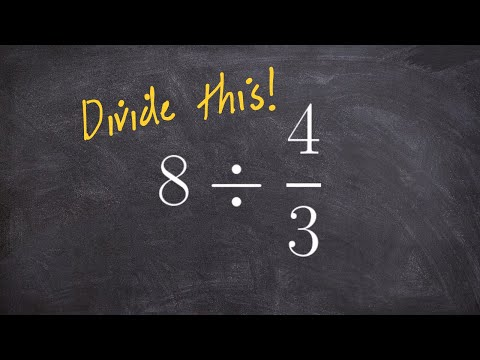By Brian Mclogan

To divide two fractions, we multiply the first fraction by the reciprocal of the second fraction.# Dividing whole numbers and fractions: t-shirts

##By Khan Academy

Dividing Fractions Word Problem. All Khan Academy content is available for free at www.khanacademy.org# 5.NF.B.7C.a. Fraction Divided by Whole Number

##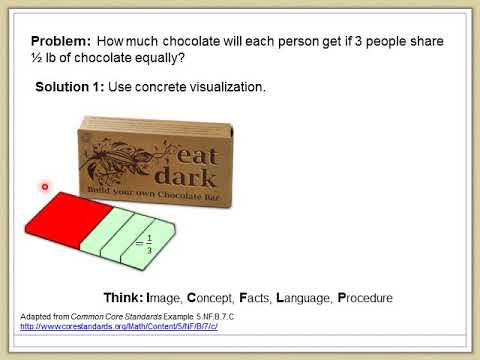By LANL MSA# Fractions 4th Grade

##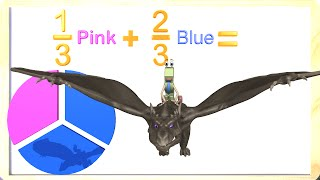By Math & Learning Videos 4 Kids

This video teaches fractions to 3rd and 4th graders. Kids will learn about fractions and how they get divided equally. A fraction represents a part of a whole. Kids will enjoy learning in this fun video with the Number One and his pet Dragon.# Dividing Mixed Numbers and Fractions

##By Khan Academy

Here is another helpful video from the Khan Academy. In this video Sal Khan clearly explains how to divide fractions when whole or mixed numbers are involved.# Dividing Whole Numbers and Applications 4

##By Khan Academy

This video lecture series on Developmental Math by Khan Academy provides developmental math examples from the Monterey Institute. These start pretty basic and would prepare a student for the Worked Examples in Algebra course lectures.....# Grade 5 Math - Division of Whole Numbers

##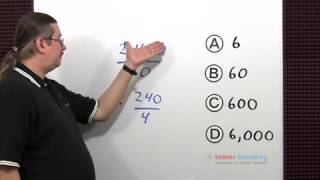By Lumos Learning

Using the Lumos Study Programs, parents and educators can reinforce the classroom learning experience for children and help them succeed at school and on the standardized tests. Lumos books, dvd, eLearning and tutoring are used by leading schools, libraries and thousands of parents to supplement classroom learning and improve student achievement in the standardized tests.# Grade 6 Math - Division of Whole Numbers

##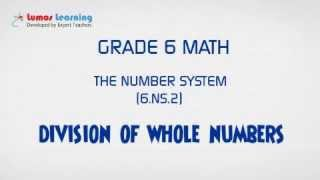By Lumos Learning

Using the Lumos Study Programs, parents and educators can reinforce the classroom learning experience for children and help them succeed at school and on the standardized tests. Lumos books, dvd, eLearning and tutoring are used by leading schools, libraries and thousands of parents to supplement classroom learning and improve student achievement in the standardized tests.